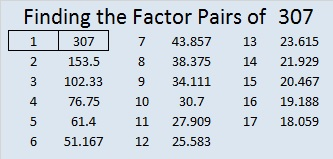# 307 and Level 5

• 307 is a prime number.
• Prime factorization: 307 is prime.
• The exponent of prime number 307 is 1. Adding 1 to that exponent we get (1 + 1) = 2. Therefore 307 has exactly 2 factors.
• Factors of 307: 1, 307
• Factor pairs: 307 = 1 x 307
• 307 has no square factors that allow its square root to be simplified. √307 ≈ 17.521How do we know that 307 is a prime number? If 307 were not a prime number, then it would be divisible by at least one prime number less than or equal to √307 ≈ 17.521. Since 307 cannot be divided evenly by 2, 3, 5, 7, 11, 13, or 17, we know that 307 is a prime number.

Eleven clues that might stump you:Print the puzzles or type the factors on this excel file: 10 Factors 2014-11-24